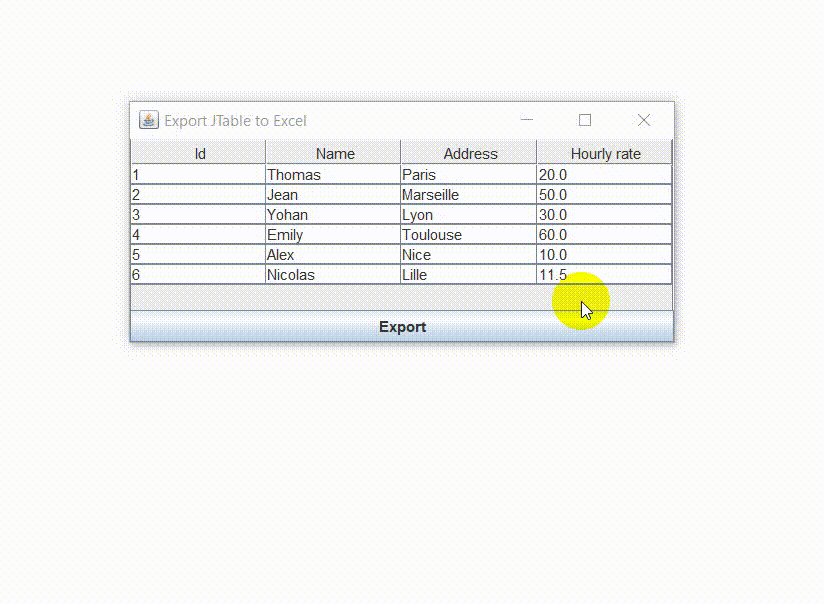Java Swing GUI

# Export Data from JTable to Excel in Java

In this tutorial, we are going to see how to export data from JTable to excel in Java. JTable is a flexible Swing component that is very well suited to display data in a tabular format.

Excel uses complicated formatting for its native .xls files but it also supports other formats. For this tutorial, we will use Tab-Separated Values (TSV), which are commonly used to transfer information from a database program to a spreadsheet.###### Java Program to Export Data from JTable to Excel in Java:
```import javax.swing.table.*;
import javax.swing.*;
import java.awt.event.*;
import java.awt.*;
import java.io.*;

class JTableToExcel extends JFrame
{
String[] columns = new String[] {
"Id",
"Name",
"Hourly rate",
};

//data for JTable in a 2D table
Object[][] data = new Object[][] {
{1, "Thomas", "Paris", 20.0 },
{2, "Jean", "Marseille", 50.0 },
{3, "Yohan", "Lyon", 30.0 },
{4, "Emily", "Toulouse", 60.0 },
{5, "Alex", "Nice", 10.0 },
{6, "Nicolas", "Lille", 11.5 },
};

//create a JTable with data
JTable table = new JTable(data, columns);
JPanel panel = new JPanel(new BorderLayout());
JButton btn = new JButton("Export");

public JTableToExcel(){
setSize(450,200);
setDefaultCloseOperation(EXIT_ON_CLOSE);
setLocationRelativeTo(null);
setTitle("Export JTable to Excel");
setVisible(true);
}

public void export(JTable table, File file){
try
{
TableModel m = table.getModel();
FileWriter fw = new FileWriter(file);

for(int i = 0; i < m.getColumnCount(); i++){
fw.write(m.getColumnName(i) + "\t");
}

fw.write("\n");

for(int i=0; i < m.getRowCount(); i++) {
for(int j=0; j < m.getColumnCount(); j++) {
fw.write(m.getValueAt(i,j).toString()+"\t");
}
fw.write("\n");
}

fw.close();
}
catch(IOException e){ System.out.println(e); }
}

public static void main(String[] args){
new JTableToExcel();
}

class MyListener implements ActionListener{
public void actionPerformed(ActionEvent e){
if(e.getSource() == btn){
JFileChooser fchoose = new JFileChooser();
int option = fchoose.showSaveDialog(JTableToExcel.this);
if(option == JFileChooser.APPROVE_OPTION){
String name = fchoose.getSelectedFile().getName();
String path = fchoose.getSelectedFile().getParentFile().getPath();
String file = path + "\\" + name + ".xls";
export(table, new File(file));
}
}
}
}
}```

###### Explanation:
`public void export(JTable table, File file){`

Here we have a method that accepts two parameters: a table where the data comes from and a file to which the data will be written.

`TableModel m = table.getModel();`

Each table object uses a table model to manage the actual table data. Thus, in this code, the model object has been defined as TableModel. The getModel() method then returns the table object’s model, which was passed in as a parameter, and then assigns it to the model object. Therefore, the model object now contains the data to be written to the Excel file.

`FileWriter fw = new FileWriter(file);`

The FileWriter class is intended for writing character streams. This line of code constructs a FileWriter object with a File object to write to.

```for(int i = 0; i < m.getColumnCount(); i++){
fw.write(m.getColumnName(i) + "\t");
}```

This loop goes through the columns, then simply writes the column name followed by a tab `("\t")` in the FileWriter object to the last column, then moves to the next line `("\n")`.

```for(int i=0; i < m.getRowCount(); i++) {
for(int j=0; j < m.getColumnCount(); j++) {
fw.write(m.getValueAt(i,j).toString()+"\t");
}
fw.write("\n");
}```

The previous loop was looping through a single row (obviously, there is only one row for column names). This time, it will iterate through a row to get the value of each column, write it to the FileWriter object followed by a tab `("\t")`, and then move to the next row `("\n")`. This process will be repeated until the last row … and this explains the need for a nested loop – the outer loop repeats what the inner loop has repeated.

`fw.close();`

Closes the data stream.MCQPractice competitive and technical Multiple Choice Questions and Answers (MCQs) with simple and logical explanations to prepare for tests and interviews.Read More

### One thought on “Export Data from JTable to Excel in Java”

•cheng